# Woodwards rule

student
Jan. 31, 2016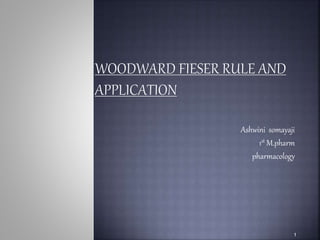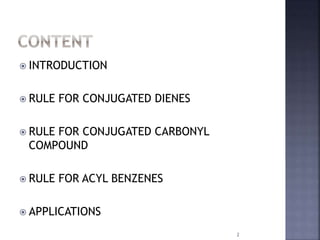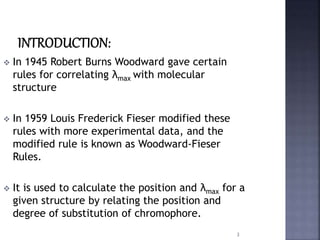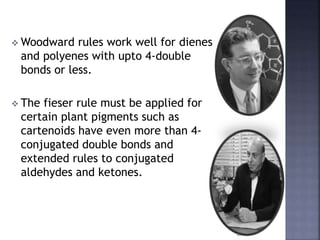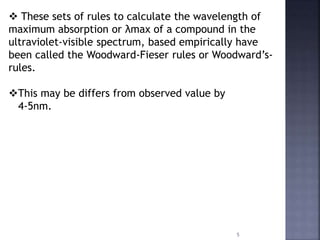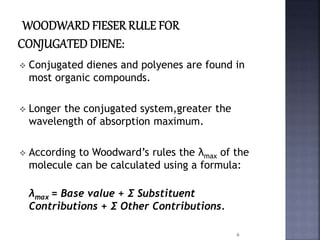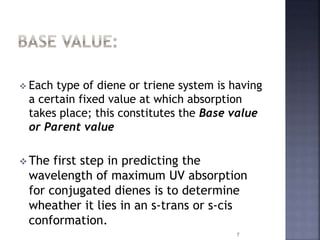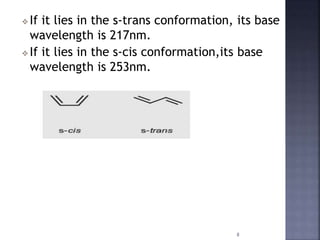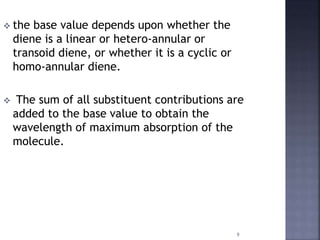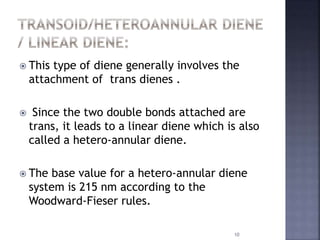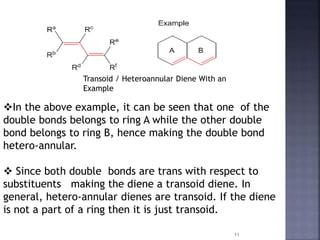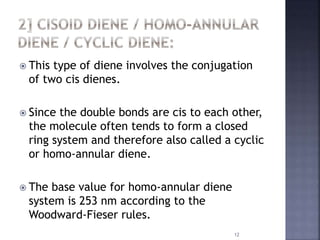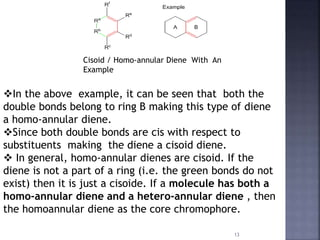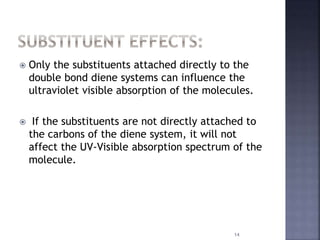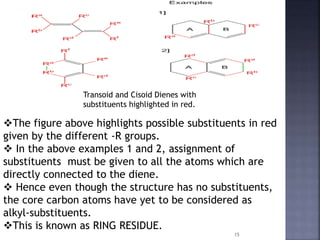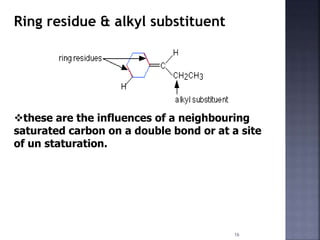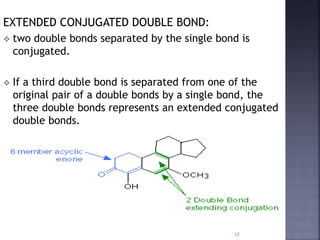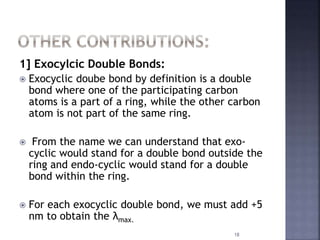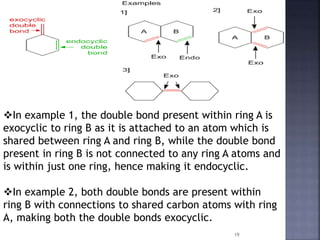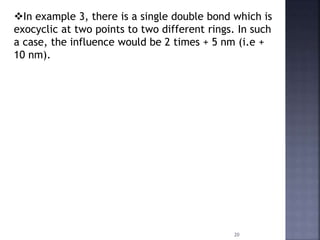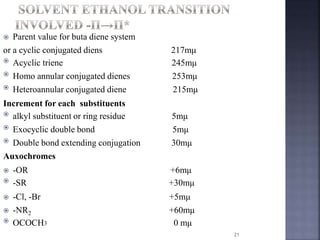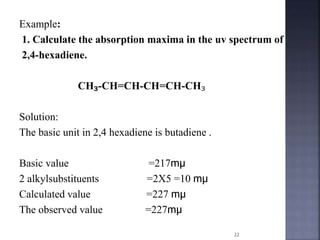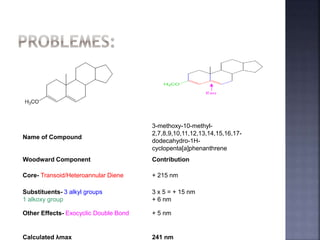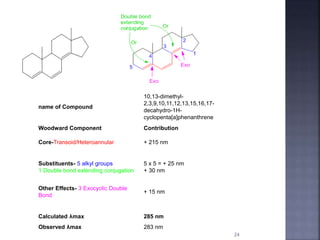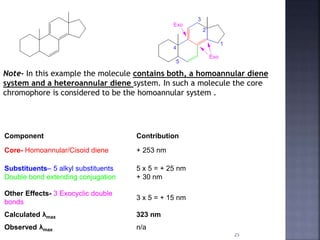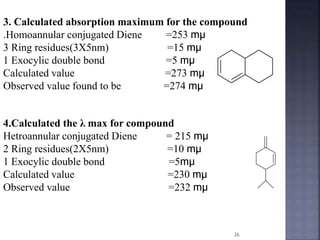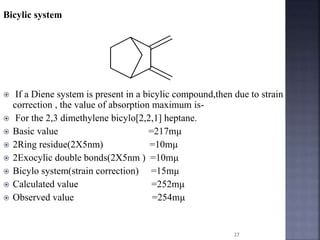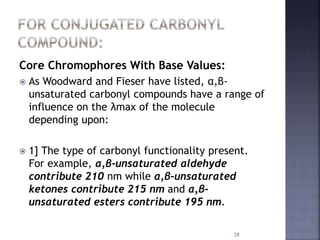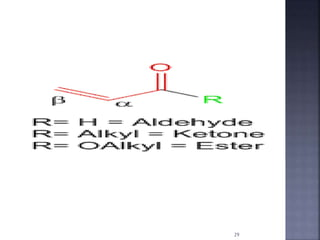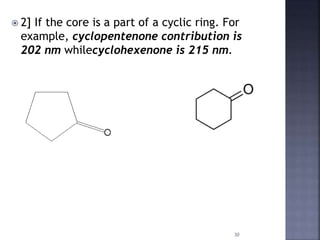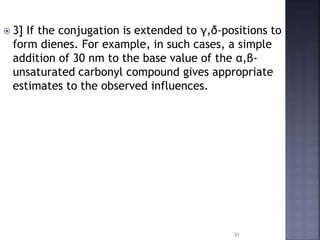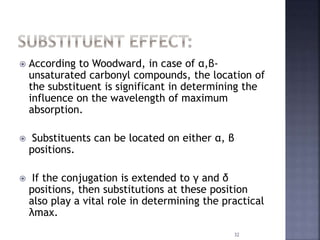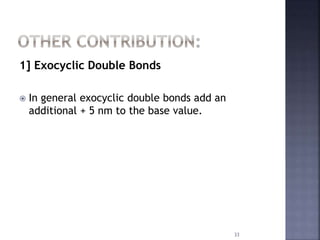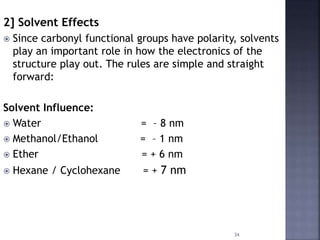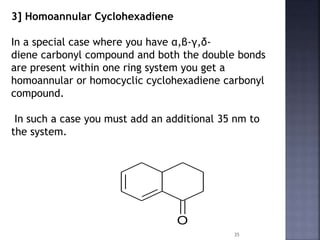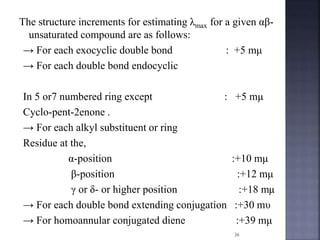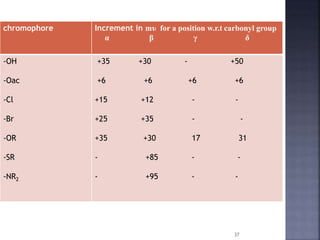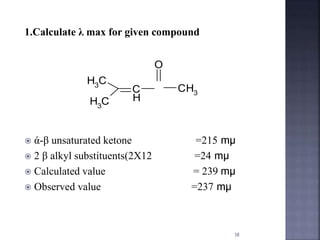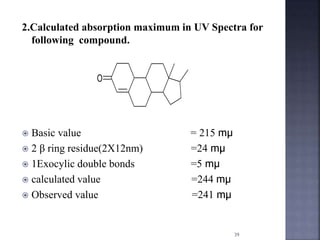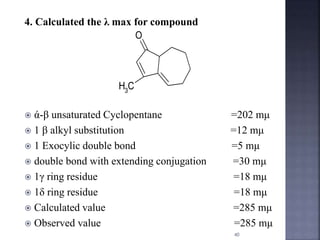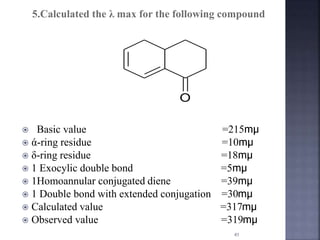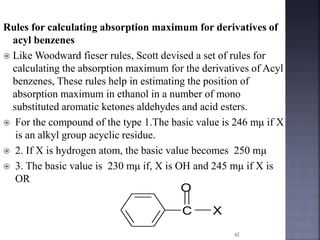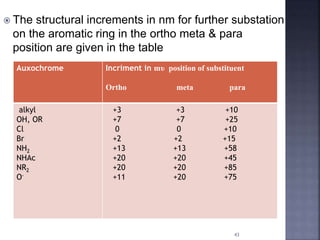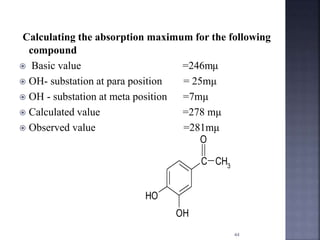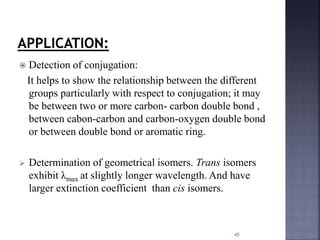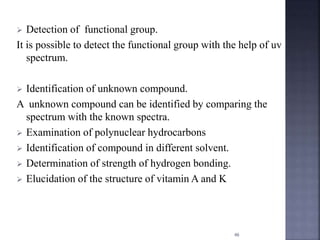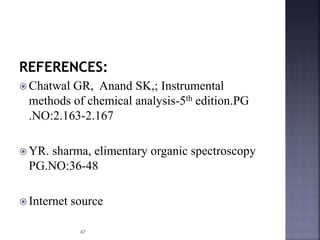1 of 48

### Woodwards rule

1. Ashwini somayaji 1st M.pharm pharmacology 1
2.  INTRODUCTION  RULE FOR CONJUGATED DIENES  RULE FOR CONJUGATED CARBONYL COMPOUND  RULE FOR ACYL BENZENES  APPLICATIONS 2
3.  In 1945 Robert Burns Woodward gave certain rules for correlating λmax with molecular structure  In 1959 Louis Frederick Fieser modified these rules with more experimental data, and the modified rule is known as Woodward-Fieser Rules.  It is used to calculate the position and λmax for a given structure by relating the position and degree of substitution of chromophore. 3
4.  Woodward rules work well for dienes and polyenes with upto 4-double bonds or less.  The fieser rule must be applied for certain plant pigments such as cartenoids have even more than 4- conjugated double bonds and extended rules to conjugated aldehydes and ketones. 4
5.  These sets of rules to calculate the wavelength of maximum absorption or λmax of a compound in the ultraviolet-visible spectrum, based empirically have been called the Woodward-Fieser rules or Woodward’s- rules. This may be differs from observed value by 4-5nm. 5
6.  Conjugated dienes and polyenes are found in most organic compounds.  Longer the conjugated system,greater the wavelength of absorption maximum.  According to Woodward’s rules the λmax of the molecule can be calculated using a formula: λmax = Base value + Σ Substituent Contributions + Σ Other Contributions. 6
7.  Each type of diene or triene system is having a certain fixed value at which absorption takes place; this constitutes the Base value or Parent value  The first step in predicting the wavelength of maximum UV absorption for conjugated dienes is to determine wheather it lies in an s-trans or s-cis conformation. 7
8. 8  If it lies in the s-trans conformation, its base wavelength is 217nm.  If it lies in the s-cis conformation,its base wavelength is 253nm.
9. 9  the base value depends upon whether the diene is a linear or hetero-annular or transoid diene, or whether it is a cyclic or homo-annular diene.  The sum of all substituent contributions are added to the base value to obtain the wavelength of maximum absorption of the molecule.
10.  This type of diene generally involves the attachment of trans dienes .  Since the two double bonds attached are trans, it leads to a linear diene which is also called a hetero-annular diene.  The base value for a hetero-annular diene system is 215 nm according to the Woodward-Fieser rules. 10
11. 11 Transoid / Heteroannular Diene With an Example In the above example, it can be seen that one of the double bonds belongs to ring A while the other double bond belongs to ring B, hence making the double bond hetero-annular.  Since both double bonds are trans with respect to substituents making the diene a transoid diene. In general, hetero-annular dienes are transoid. If the diene is not a part of a ring then it is just transoid.
12.  This type of diene involves the conjugation of two cis dienes.  Since the double bonds are cis to each other, the molecule often tends to form a closed ring system and therefore also called a cyclic or homo-annular diene.  The base value for homo-annular diene system is 253 nm according to the Woodward-Fieser rules. 12
13. 13 Cisoid / Homo-annular Diene With An Example In the above example, it can be seen that both the double bonds belong to ring B making this type of diene a homo-annular diene. Since both double bonds are cis with respect to substituents making the diene a cisoid diene.  In general, homo-annular dienes are cisoid. If the diene is not a part of a ring (i.e. the green bonds do not exist) then it is just a cisoide. If a molecule has both a homo-annular diene and a hetero-annular diene , then the homoannular diene as the core chromophore.
14.  Only the substituents attached directly to the double bond diene systems can influence the ultraviolet visible absorption of the molecules.  If the substituents are not directly attached to the carbons of the diene system, it will not affect the UV-Visible absorption spectrum of the molecule. 14
15. 15 Transoid and Cisoid Dienes with substituents highlighted in red. The figure above highlights possible substituents in red given by the different -R groups.  In the above examples 1 and 2, assignment of substituents must be given to all the atoms which are directly connected to the diene.  Hence even though the structure has no substituents, the core carbon atoms have yet to be considered as alkyl-substituents. This is known as RING RESIDUE.
16. 16 Ring residue & alkyl substituent these are the influences of a neighbouring saturated carbon on a double bond or at a site of un staturation.
17. 17 EXTENDED CONJUGATED DOUBLE BOND:  two double bonds separated by the single bond is conjugated.  If a third double bond is separated from one of the original pair of a double bonds by a single bond, the three double bonds represents an extended conjugated double bonds.
18. 1] Exocylcic Double Bonds:  Exocyclic doube bond by definition is a double bond where one of the participating carbon atoms is a part of a ring, while the other carbon atom is not part of the same ring.  From the name we can understand that exo- cyclic would stand for a double bond outside the ring and endo-cyclic would stand for a double bond within the ring.  For each exocyclic double bond, we must add +5 nm to obtain the λmax. 18
19. 19 In example 1, the double bond present within ring A is exocyclic to ring B as it is attached to an atom which is shared between ring A and ring B, while the double bond present in ring B is not connected to any ring A atoms and is within just one ring, hence making it endocyclic. In example 2, both double bonds are present within ring B with connections to shared carbon atoms with ring A, making both the double bonds exocyclic.
20. 20 In example 3, there is a single double bond which is exocyclic at two points to two different rings. In such a case, the influence would be 2 times + 5 nm (i.e + 10 nm).
21.  Parent value for buta diene system or a cyclic conjugated diens 217mμ  Acyclic triene 245mμ  Homo annular conjugated dienes 253mμ  Heteroannular conjugated diene 215mμ Increment for each substituents  alkyl substituent or ring residue 5mμ  Exocyclic double bond 5mμ  Double bond extending conjugation 30mμ Auxochromes  -OR +6mμ  -SR +30mμ  -Cl, -Br +5mμ  -NR2 +60mμ  OCOCH3 0 mμ 21
22. Example: 1. Calculate the absorption maxima in the uv spectrum of 2,4-hexadiene. CH₃-CH=CH-CH=CH-CH₃ Solution: The basic unit in 2,4 hexadiene is butadiene . Basic value =217mμ 2 alkylsubstituents =2X5 =10 mμ Calculated value =227 mμ The observed value =227mμ 22
23. 23 Name of Compound 3-methoxy-10-methyl- 2,7,8,9,10,11,12,13,14,15,16,17- dodecahydro-1H- cyclopenta[a]phenanthrene Woodward Component Contribution Core- Transoid/Heteroannular Diene + 215 nm Substituents- 3 alkyl groups 1 alkoxy group 3 x 5 = + 15 nm + 6 nm Other Effects- Exocyclic Double Bond + 5 nm Calculated λmax 241 nm
24. 24 name of Compound 10,13-dimethyl- 2,3,9,10,11,12,13,15,16,17- decahydro-1H- cyclopenta[a]phenanthrene Woodward Component Contribution Core-Transoid/Heteroannular + 215 nm Substituents- 5 alkyl groups 1 Double bond extending conjugation 5 x 5 = + 25 nm + 30 nm Other Effects- 3 Exocyclic Double Bond + 15 nm Calculated λmax 285 nm Observed λmax 283 nm
25. 25 Note- In this example the molecule contains both, a homoannular diene system and a heteroannular diene system. In such a molecule the core chromophore is considered to be the homoannular system . Component Contribution Core- Homoannular/Cisoid diene + 253 nm Substituents– 5 alkyl substituents Double bond extending conjugation 5 x 5 = + 25 nm + 30 nm Other Effects- 3 Exocyclic double bonds 3 x 5 = + 15 nm Calculated λmax 323 nm Observed λmax n/a
26. 3. Calculated absorption maximum for the compound .Homoannular conjugated Diene =253 mμ 3 Ring residues(3X5nm) =15 mμ 1 Exocylic double bond =5 mμ Calculated value =273 mμ Observed value found to be =274 mμ 4.Calculated the λ max for compound Hetroannular conjugated Diene = 215 mμ 2 Ring residues(2X5nm) =10 mμ 1 Exocylic double bond =5mμ Calculated value =230 mμ Observed value =232 mμ 26
27. Bicylic system  If a Diene system is present in a bicylic compound,then due to strain correction , the value of absorption maximum is-  For the 2,3 dimethylene bicylo[2,2,1] heptane.  Basic value =217mμ  2Ring residue(2X5nm) =10mμ  2Exocylic double bonds(2X5nm ) =10mμ  Bicylo system(strain correction) =15mμ  Calculated value =252mμ  Observed value =254mμ 27
28. 28 Core Chromophores With Base Values:  As Woodward and Fieser have listed, α,β- unsaturated carbonyl compounds have a range of influence on the λmax of the molecule depending upon:  1] The type of carbonyl functionality present. For example, α,β-unsaturated aldehyde contribute 210 nm while α,β-unsaturated ketones contribute 215 nm and α,β- unsaturated esters contribute 195 nm.
29. 29
30. 30  2] If the core is a part of a cyclic ring. For example, cyclopentenone contribution is 202 nm whilecyclohexenone is 215 nm.
31. 31  3] If the conjugation is extended to γ,δ-positions to form dienes. For example, in such cases, a simple addition of 30 nm to the base value of the α,β- unsaturated carbonyl compound gives appropriate estimates to the observed influences.
32.  According to Woodward, in case of α,β- unsaturated carbonyl compounds, the location of the substituent is significant in determining the influence on the wavelength of maximum absorption.  Substituents can be located on either α, β positions.  If the conjugation is extended to γ and δ positions, then substitutions at these position also play a vital role in determining the practical λmax. 32
33. 1] Exocyclic Double Bonds  In general exocyclic double bonds add an additional + 5 nm to the base value. 33
34. 34 2] Solvent Effects  Since carbonyl functional groups have polarity, solvents play an important role in how the electronics of the structure play out. The rules are simple and straight forward: Solvent Influence:  Water = – 8 nm  Methanol/Ethanol = – 1 nm  Ether = + 6 nm  Hexane / Cyclohexane = + 7 nm
35. 35 3] Homoannular Cyclohexadiene In a special case where you have α,β-γ,δ- diene carbonyl compound and both the double bonds are present within one ring system you get a homoannular or homocyclic cyclohexadiene carbonyl compound. In such a case you must add an additional 35 nm to the system. O
36. The structure increments for estimating λmax for a given αβ- unsaturated compound are as follows: → For each exocyclic double bond : +5 mμ → For each double bond endocyclic In 5 or7 numbered ring except : +5 mμ Cyclo-pent-2enone . → For each alkyl substituent or ring Residue at the, α-position :+10 mμ β-position :+12 mμ γ or δ- or higher position :+18 mμ → For each double bond extending conjugation :+30 mυ → For homoannular conjugated diene :+39 mμ 36
37. chromophore Increment in mυ for a position w.r.t carbonyl group α β γ δ -OH -Oac -Cl -Br -OR -SR -NR2 +35 +30 - +50 +6 +6 +6 +6 +15 +12 - - +25 +35 - - +35 +30 17 31 - +85 - - - +95 - - 37
38. 1.Calculate λ max for given compound  ά-β unsaturated ketone =215 mμ  2 β alkyl substituents(2X12 =24 mμ  Calculated value = 239 mμ  Observed value =237 mμ CH3 CH3 C H O CH3 38
39. 2.Calculated absorption maximum in UV Spectra for following compound.  Basic value = 215 mμ  2 β ring residue(2X12nm) =24 mμ  1Exocylic double bonds =5 mμ  calculated value =244 mμ  Observed value =241 mμ O 39
40. 4. Calculated the λ max for compound  ά-β unsaturated Cyclopentane =202 mμ  1 β alkyl substitution =12 mμ  1 Exocylic double bond =5 mμ  double bond with extending conjugation =30 mμ  1γ ring residue =18 mμ  1δ ring residue =18 mμ  Calculated value =285 mμ  Observed value =285 mμ O CH3 40
41. 5.Calculated the λ max for the following compound  Basic value =215mμ  ά-ring residue =10mμ  δ-ring residue =18mμ  1 Exocylic double bond =5mμ  1Homoannular conjugated diene =39mμ  1 Double bond with extended conjugation =30mμ  Calculated value =317mμ  Observed value =319mμ O 41
42. Rules for calculating absorption maximum for derivatives of acyl benzenes  Like Woodward fieser rules, Scott devised a set of rules for calculating the absorption maximum for the derivatives of Acyl benzenes, These rules help in estimating the position of absorption maximum in ethanol in a number of mono substituted aromatic ketones aldehydes and acid esters.  For the compound of the type 1.The basic value is 246 mμ if X is an alkyl group acyclic residue.  2. If X is hydrogen atom, the basic value becomes 250 mμ  3. The basic value is 230 mμ if, X is OH and 245 mμ if X is OR C O X 42
43.  The structural increments in nm for further substation on the aromatic ring in the ortho meta & para position are given in the table Auxochrome Incriment in mυ position of substituent Ortho meta para alkyl OH, OR Cl Br NH2 NHAc NR2 O- +3 +3 +10 +7 +7 +25 0 0 +10 +2 +2 +15 +13 +13 +58 +20 +20 +45 +20 +20 +85 +11 +20 +75 43
44. Calculating the absorption maximum for the following compound  Basic value =246mμ  OH- substation at para position = 25mμ  OH - substation at meta position =7mμ  Calculated value =278 mμ  Observed value =281mμ C OH OH CH3 O 44
45.  Detection of conjugation: It helps to show the relationship between the different groups particularly with respect to conjugation; it may be between two or more carbon- carbon double bond , between cabon-carbon and carbon-oxygen double bond or between double bond or aromatic ring.  Determination of geometrical isomers. Trans isomers exhibit λmax at slightly longer wavelength. And have larger extinction coefficient than cis isomers. 45
46.  Detection of functional group. It is possible to detect the functional group with the help of uv spectrum.  Identification of unknown compound. A unknown compound can be identified by comparing the spectrum with the known spectra.  Examination of polynuclear hydrocarbons  Identification of compound in different solvent.  Determination of strength of hydrogen bonding.  Elucidation of the structure of vitamin A and K 46
47. REFERENCES:  Chatwal GR, Anand SK,; Instrumental methods of chemical analysis-5th edition.PG .NO:2.163-2.167  YR. sharma, elimentary organic spectroscopy PG.NO:36-48  Internet source 47
48. 48